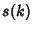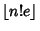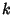## Schur's Lemma

For eachthere exists a largest Integer(known as the Schur Number) such that no matter how the set of Integers less than(whereis the Floor Function) is partitioned intoclasses, one class must contain Integers,,such that, whereandare not necessarily distinct. The upper bound has since been slightly improved to.

Guy, R. K. Schur's Problem. Partitioning Integers into Sum-Free Classes'' and The Modular Version of Schur's Problem.'' §E11 and E12 in Unsolved Problems in Number Theory, 2nd ed. New York: Springer-Verlag, pp. 209-212, 1994.